# Simulating study data

#### 2018-09-14

Simulation using simstudy has two primary steps. First, the user defines the data elements of a data set. Second, the user generates the data, using the definitions in the first step. Additional functionality exists to simulate observed or randomized treatment assignment/exposures, to generate survival data, to create longitudinal/panel data, to create multi-level/hierarchical data, to create datasets with correlated variables based on a specified covariance structure, to merge datasets, to create data sets with missing data, and to create non-linear relationships with underlying spline curves.

## Defining the Data

The key to simulating data in simstudy is the creation of series of data definition tables that look like this:

varname formula variance dist link
nr 7 0e+00 nonrandom identity
x1 10;20 0e+00 uniform identity
y1 nr + x1 * 2 8e+00 normal identity
y2 nr - 0.2 * x1 0e+00 poisson log
xnb nr - 0.2 * x1 5e-02 negBinomial log
xCat 0.3;0.2;0.5 0e+00 categorical identity
g1 5+xCat 1e+00 gamma log
b1 1+0.3*xCat 1e+00 beta logit
a1 -3 + xCat 0e+00 binary logit
a2 -3 + xCat 1e+02 binomial logit

These definition tables can be generated two ways. One option is to to use any external editor that allows the creation of csv files, which can be read in with a call to defRead. An alternative is to make repeated calls to the function defData. Here, we illustrate the R code that builds this definition table internally:

def <- defData(varname = "nr", dist = "nonrandom", formula = 7, id = "idnum")
def <- defData(def, varname = "x1", dist = "uniform", formula = "10;20")
def <- defData(def, varname = "y1", formula = "nr + x1 * 2", variance = 8)
def <- defData(def, varname = "y2", dist = "poisson", formula = "nr - 0.2 * x1",
def <- defData(def, varname = "xnb", dist = "negBinomial", formula = "nr - 0.2 * x1",
variance = 0.05, link = "log")
def <- defData(def, varname = "xCat", formula = "0.3;0.2;0.5", dist = "categorical")
def <- defData(def, varname = "g1", dist = "gamma", formula = "5+xCat", variance = 1,
def <- defData(def, varname = "b1", dist = "beta", formula = "1+0.3*xCat", variance = 1,
def <- defData(def, varname = "a1", dist = "binary", formula = "-3 + xCat",
def <- defData(def, varname = "a2", dist = "binomial", formula = "-3 + xCat",
variance = 100, link = "logit")

The first call to defData without specifying a definition name (in this example the definition name is def) creates a new data.table with a single row. An additional row is added to the table def each time the function defData is called. Each of these calls is the definition of a new field in the data set that will be generated. In this example, the first data field is named ‘nr’, defined as a constant with a value to be 7. In each call to defData the user defines a variable name, a distribution (the default is ‘normal’), a mean formula (if applicable), a variance parameter (if applicable), and a link function for the mean (defaults to ‘identity’).

The possible distributions include normal, gamma, poisson, zero-truncated poisson, negative binomial, binary, binomial, beta, uniform, uniform integer, categorical, and deterministic/non-random. For all of these distributions, key parameters defining the distribution are entered in the formula, variance, and link fields.

In the case of the normal, gamma, beta, and negative binomial distributions, the formula specifies the mean. The formula can be a scalar value (number) or a string that represents a function of previously defined variables in the data set definition (or, as we will see later, in a previously generated data set). In the example, the mean of y1, a normally distributed value, is declared as a linear function of nr and x1, and the mean of g1 is a function of the category defined by xCat. The variance field is defined only for normal, gamma, beta, and negative binomial random variables, and can only be defined as a scalar value. In the case of gamma, beta, and negative binomial variables, the value entered in variance field is really a dispersion value $$d$$. The variance of a gamma distributed variable will be $$d \times mean^2$$, for a beta distributed variable will be $$mean \times (1- mean)/(1 + d)$$, and for a negative binomial distributed variable, the variance will be $$mean + d*mean^2$$.

In the case of the poisson, zero-truncated poisson, and binary distributions, the formula also specifies the mean. The variance is not a valid parameter in these cases, but the link field is. The default link is ‘identity’ but a ‘log’ link is available for the Poisson distributions and a “logit” link is available for the binary outcomes. In this example, y2 is defined as Poisson random variable with a mean that is function of nr and x1 on the log scale. For binary variables, which take a value of 0 or 1, the formula represents probability (with the ‘identity’ link) or log odds (with the ‘logit’ link) of the variable having a value of 1. In the example, a1 has been defined as a binary random variable with a log odds that is a function of xCat.

In the case of the binomial distribution, the formula specifies the probability of success $$p$$, and the variance field is used to specify the number of trials $$n$$. The mean of this distribution is $$n*p$$, and the variance is $$n*p*(1-p)$$.

Variables defined with a uniform, uniform integer, categorical, or deterministic/non-random distribution are specified using the formula only. The variance and link fields are not used in these cases.

For a uniformly distributed variable, The formula is a string with the format “a;b”, where a and b are scalars or functions of previously defined variables. The uniform distribution has two parameters - the minimum and the maximum. In this case, a represents the minimum and b represents the maximum.

For a categorical variable with $$k$$ categories, the formula is a string of probabilities that sum to 1: “$$p_1 ; p_2 ; ... ; p_k$$”. $$p_1$$ is the probability of the random variable falling category 1, $$p_2$$ is the probability of category 2, etc. The probabilities can be specified as functions of other variables previously defined. In the example, xCat has three possibilities with probabilities 0.3, 0.2, and 0.5, respectively.

Non-random variables are defined by the formula. Since these variables are deterministic, variance is not relevant. They can be functions of previously defined variables or a scalar, as we see in the sample for variable defined as nr.

## Generating the Data

After the data set definitions have been created, a new data set with $$n$$ observations can be created with a call to function genData. In this example, 1,000 observations are generated using the data set definitions in def, and then stored in the object dt:

dt <- genData(1000, def)
dt
##       idnum nr       x1       y1  y2 xnb xCat        g1        b1 a1 a2
##    1:     1  7 18.71470 48.13110  25  36    3  882.3611 0.9707256  1 48
##    2:     2  7 12.63977 34.82680  87  97    3 1986.9499 0.8497208  1 54
##    3:     3  7 13.21247 34.96022  80  71    2 1460.2205 0.7136439  0 26
##    4:     4  7 19.21613 38.93975  17  16    1   77.6381 0.9997095  0 14
##    5:     5  7 10.70988 24.16021 148 110    2  696.4741 0.9546023  0 32
##   ---
##  996:   996  7 12.69114 34.43474  88 117    1  480.9245 0.3714678  0 10
##  997:   997  7 11.48129 31.34903 108 125    1  235.4808 0.9427933  0 13
##  998:   998  7 16.88184 41.60436  45  50    3 2425.0456 0.9999994  1 51
##  999:   999  7 10.24263 25.36589 151 107    3 2537.5048 0.9978473  0 49
## 1000:  1000  7 12.72076 33.53079  78  70    1  605.9685 0.4719441  0 13

New data can be added to an existing data set with a call to function addColumns. The new data definitions are created with a call to defData and then included as an argument in the call to addColumns:

addef <- defDataAdd(varname = "zExtra", dist = "normal", formula = "3 + y1",
variance = 2)

dt
##       idnum nr       x1       y1  y2 xnb xCat        g1        b1 a1 a2
##    1:     1  7 18.71470 48.13110  25  36    3  882.3611 0.9707256  1 48
##    2:     2  7 12.63977 34.82680  87  97    3 1986.9499 0.8497208  1 54
##    3:     3  7 13.21247 34.96022  80  71    2 1460.2205 0.7136439  0 26
##    4:     4  7 19.21613 38.93975  17  16    1   77.6381 0.9997095  0 14
##    5:     5  7 10.70988 24.16021 148 110    2  696.4741 0.9546023  0 32
##   ---
##  996:   996  7 12.69114 34.43474  88 117    1  480.9245 0.3714678  0 10
##  997:   997  7 11.48129 31.34903 108 125    1  235.4808 0.9427933  0 13
##  998:   998  7 16.88184 41.60436  45  50    3 2425.0456 0.9999994  1 51
##  999:   999  7 10.24263 25.36589 151 107    3 2537.5048 0.9978473  0 49
## 1000:  1000  7 12.72076 33.53079  78  70    1  605.9685 0.4719441  0 13
##         zExtra
##    1: 53.34158
##    2: 35.55258
##    3: 39.74581
##    4: 38.61562
##    5: 27.66564
##   ---
##  996: 39.48614
##  997: 35.11438
##  998: 43.95011
##  999: 27.75513
## 1000: 38.88947

## Generating the Treatment/Exposure

Treatment assignment can be accomplished through the original data generation process, using defData and genData. However, the functions trtAssign and trtObserve provide more options to generate treatment assignment.

### Assigned treatment

Treatment assignment can simulate how treatment is made in a randomized study. Assignment to treatment groups can be (close to) balanced (as would occur in a block randomized trial); this balancing can be done without or without strata. Alternatively, the assignment can be left to chance without blocking; in this case, balance across treatment groups is not guaranteed, particularly with small sample sizes.

First, create the data definition:

def <- defData(varname = "male", dist = "binary", formula = 0.5, id = "cid")
def <- defData(def, varname = "over65", dist = "binary", formula = "-1.7 + .8*male",
def <- defData(def, varname = "baseDBP", dist = "normal", formula = 70, variance = 40)

dtstudy <- genData(330, def)

Balanced treatment assignment, stratified by gender and age category (not blood pressure)

study1 <- trtAssign(dtstudy, n = 3, balanced = TRUE, strata = c("male", "over65"),
grpName = "rxGrp")

study1
##      cid rxGrp male over65  baseDBP
##   1:   1     3    1      0 69.71811
##   2:   2     1    0      0 68.21481
##   3:   3     3    1      0 63.64589
##   4:   4     2    0      0 67.40492
##   5:   5     3    0      0 72.96366
##  ---
## 326: 326     1    1      1 67.99205
## 327: 327     3    1      0 55.73555
## 328: 328     3    1      0 74.85385
## 329: 329     3    1      0 75.20651
## 330: 330     3    1      0 66.24796

Balanced treatment assignment (without stratification)

study2 <- trtAssign(dtstudy, n = 3, balanced = TRUE, grpName = "rxGrp")

Random (unbalanced) treatment assignment

study3 <- trtAssign(dtstudy, n = 3, balanced = FALSE, grpName = "rxGrp")

Comparison of three treatment assignment mechanisms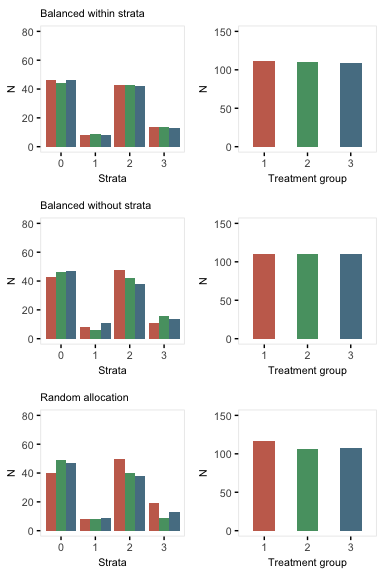### Observed treatment

If exposure or treatment is observed (rather than randomly assigned), use trtObserve to generate groups. There may be any number of possible exposure or treatment groups, and the probability of exposure to a specific level can depend on covariates already in the data set. In this case, there are three exposure groups that vary by gender and age:

formula1 <- c("-2 + 2*male - .5*over65", "-1 + 2*male + .5*over65")
dtExp <- trtObserve(dtstudy, formulas = formula1, logit.link = TRUE, grpName = "exposure")

Here are the exposure distributions by gender and age: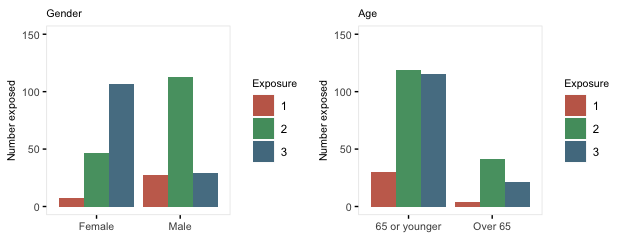Here is a second case of three exposures where the exposure is independent of any covariates. Note that specifying the formula as c(.35, .45) is the same as specifying it is c(.35, .45, .20). Also, when referring to probabilities, the identity link is used:

formula2 <- c(0.35, 0.45)

dtExp2 <- trtObserve(dtstudy, formulas = formula2, logit.link = FALSE, grpName = "exposure")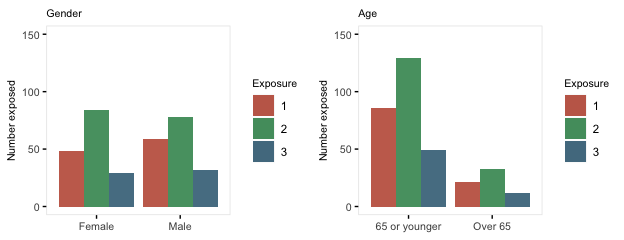## Survival Data

Time-to-event data, including both survival and censoring times, are created using functions defSurv and genSurv. The survival data definitions require a variable name as well as a specification of a scale value, which determines the mean survival time at a baseline level of covariates (i.e. all covariates set to 0). The Weibull distribution is used to generate these survival times. In addition, covariates (which have been defined previously) that influence survival time can be included in the formula field. Positive coefficients are associated with longer survival times (and lower hazard rates). Finally, the shape of the distribution can be specified. A shape value of 1 reflects the exponential distribution.

# Baseline data definitions

def <- defData(varname = "x1", formula = 0.5, dist = "binary")
def <- defData(def, varname = "x2", formula = 0.5, dist = "binary")
def <- defData(def, varname = "grp", formula = 0.5, dist = "binary")

# Survival data definitions

sdef <- defSurv(varname = "survTime", formula = "1.5*x1", scale = "grp*50 + (1-grp)*25",
shape = "grp*1 + (1-grp)*1.5")
sdef <- defSurv(sdef, varname = "censorTime", scale = 80, shape = 1)

sdef
##       varname formula               scale               shape
## 1:   survTime  1.5*x1 grp*50 + (1-grp)*25 grp*1 + (1-grp)*1.5
## 2: censorTime       0                  80                   1

The data are generated with calls to genData and genSurv:

# Baseline data definitions

dtSurv <- genData(300, def)
dtSurv <- genSurv(dtSurv, sdef)

head(dtSurv)
##    id x1 x2 grp survTime censorTime
## 1:  1  0  1   0  201.739     53.291
## 2:  2  1  1   1    3.178     79.894
## 3:  3  0  1   0   18.586     90.442
## 4:  4  1  0   0    8.069     94.643
## 5:  5  1  0   0    1.354     14.280
## 6:  6  1  0   1   12.198      2.611
# A comparison of survival by group and x1

dtSurv[, round(mean(survTime), 1), keyby = .(grp, x1)]
##    grp x1    V1
## 1:   0  0 149.7
## 2:   0  1  16.0
## 3:   1  0  48.8
## 4:   1  1  10.4

Observed survival times and censoring indicators can be generated by defining new fields:

cdef <- defDataAdd(varname = "obsTime", formula = "pmin(survTime, censorTime)",
dist = "nonrandom")
cdef <- defDataAdd(cdef, varname = "status", formula = "I(survTime <= censorTime)",
dist = "nonrandom")

dtSurv <- addColumns(cdef, dtSurv)

head(dtSurv)
##    id x1 x2 grp survTime censorTime obsTime status
## 1:  1  0  1   0  201.739     53.291  53.291      0
## 2:  2  1  1   1    3.178     79.894   3.178      1
## 3:  3  0  1   0   18.586     90.442  18.586      1
## 4:  4  1  0   0    8.069     94.643   8.069      1
## 5:  5  1  0   0    1.354     14.280   1.354      1
## 6:  6  1  0   1   12.198      2.611   2.611      0
# estimate proportion of censoring by x1 and group

dtSurv[, round(1 - mean(status), 2), keyby = .(grp, x1)]
##    grp x1   V1
## 1:   0  0 0.62
## 2:   0  1 0.12
## 3:   1  0 0.30
## 4:   1  1 0.14

Here is a Kaplan-Meier plot of the data by the four groups: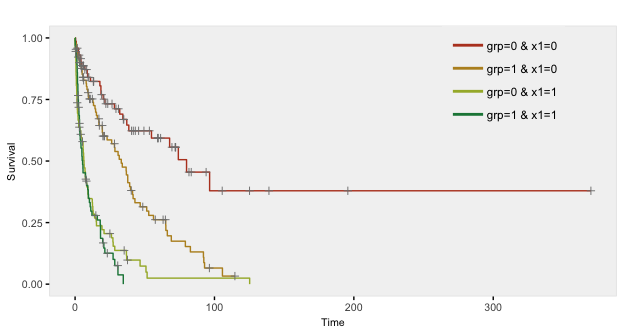## Longitudinal Data

To simulate longitudinal data, we start with a ‘cross-sectional’ data set and convert it to a time-dependent data set. The original cross-sectional data set may or may not include time-dependent data in the columns. In the next example, we measure outcome Y once before and twice after intervention T in a randomized trial:

tdef <- defData(varname = "T", dist = "binary", formula = 0.5)
tdef <- defData(tdef, varname = "Y0", dist = "normal", formula = 10, variance = 1)
tdef <- defData(tdef, varname = "Y1", dist = "normal", formula = "Y0 + 5 + 5 * T",
variance = 1)
tdef <- defData(tdef, varname = "Y2", dist = "normal", formula = "Y0 + 10 + 5 * T",
variance = 1)

dtTrial <- genData(500, tdef)
dtTrial
##       id T        Y0       Y1       Y2
##   1:   1 1  9.445490 21.03971 24.94071
##   2:   2 0 10.637504 17.11626 20.81806
##   3:   3 0 10.112860 12.84198 21.53933
##   4:   4 0  9.775861 14.03469 18.03353
##   5:   5 0 10.023485 13.11393 19.35539
##  ---
## 496: 496 1  9.515709 18.62110 24.78710
## 497: 497 0  9.423493 16.10887 20.81375
## 498: 498 0 10.046107 14.65857 19.62253
## 499: 499 0 11.417567 15.99290 21.31755
## 500: 500 0 10.031238 15.69252 21.06535

The data in longitudinal form is created with a call to addPeriods. If the cross-sectional data includes time dependent data, then the number of periods nPeriods must be the same as the number of time dependent columns. If a variable is not declared as one of the timevars, it will be repeated each time period. In this example, the treatment indicator T is not specified as a time dependent variable. (Note: if there are two time-dependent variables, it is best to create two data sets and merge them. This will be shown later in the vignette).

dtTime <- addPeriods(dtTrial, nPeriods = 3, idvars = "id", timevars = c("Y0",
"Y1", "Y2"), timevarName = "Y")
dtTime
##        id period T        Y timeID
##    1:   1      0 1  9.44549      1
##    2:   1      1 1 21.03971      2
##    3:   1      2 1 24.94071      3
##    4:   2      0 0 10.63750      4
##    5:   2      1 0 17.11626      5
##   ---
## 1496: 499      1 0 15.99290   1496
## 1497: 499      2 0 21.31755   1497
## 1498: 500      0 0 10.03124   1498
## 1499: 500      1 0 15.69252   1499
## 1500: 500      2 0 21.06535   1500

This is what the longitudinal data look like: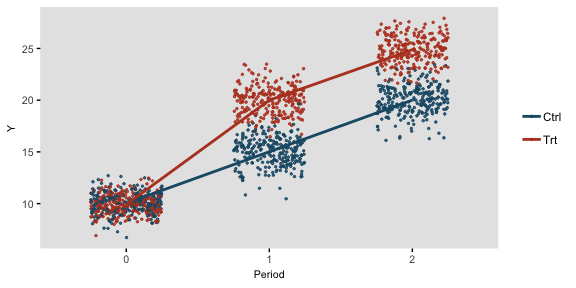### Longitudinal data with varying observation and interval times

It is also possible to generate longitudinal data with varying numbers of measurement periods as well as varying time intervals between each measurement period. This is done by defining specific variables in the data set that define the number of observations per subject and the average interval time between each observation. nCount defines the number of measurements for an individual; mInterval specifies the average time between intervals for an subject; and vInterval specifies the variance of those interval times. If vInterval is set to 0 or is not defined, the interval for a subject is determined entirely by the mean interval. If vInterval is greater than 0, time intervals are generated using a gamma distribution with mean and dispersion specified.

In this simple example, the cross-sectional data generates individuals with a different number of measurement observations and different times between each observation. Data for two of these individuals is printed:

def <- defData(varname = "xbase", dist = "normal", formula = 20, variance = 3)
def <- defData(def, varname = "nCount", dist = "noZeroPoisson", formula = 6)
def <- defData(def, varname = "mInterval", dist = "gamma", formula = 30, variance = 0.01)
def <- defData(def, varname = "vInterval", dist = "nonrandom", formula = 0.07)

dt <- genData(200, def)
dt[id %in% c(8, 121)]  # View individuals 8 and 121
##     id    xbase nCount mInterval vInterval
## 1:   8 18.62292      6  21.82117      0.07
## 2: 121 17.59532      3  33.93970      0.07

The resulting longitudinal data for these two subjects can be inspected after a call to addPeriods. Notice that no parameters need to be set since all information resides in the data set itself:

dtPeriod <- addPeriods(dt)
dtPeriod[id %in% c(8, 121)]  # View individuals 8 and 121 only
##     id period    xbase time timeID
## 1:   8      0 18.62292    0     50
## 2:   8      1 18.62292   12     51
## 3:   8      2 18.62292   26     52
## 4:   8      3 18.62292   52     53
## 5:   8      4 18.62292   76     54
## 6:   8      5 18.62292  101     55
## 7: 121      0 17.59532    0    696
## 8: 121      1 17.59532   32    697
## 9: 121      2 17.59532   69    698

If a time sensitive measurement is added to the data set …

def2 <- defDataAdd(varname = "Y", dist = "normal", formula = "15 + .1 * time",
variance = 5)
dtPeriod <- addColumns(def2, dtPeriod)

… a plot of a five randomly selected individuals looks like this: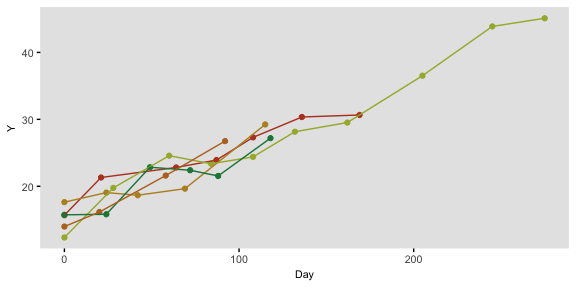## Clustered Data

The function genCluster generates multilevel or clustered data based on a previously generated data set that is one “level” up from the clustered data. For example, if there is a data set that contains school level (considered here to be level 2), classrooms (level 1) can be generated. And then, students (now level 1) can be generated within classrooms (now level 2)

In the example here, we do in fact generate school, class, and student level data. There are eight schools, four of which are randomized to receive an intervention. The number of classes per school varies, as does the number of students per class. (It is straightforward to generate fully balanced data by using constant values.) The outcome of interest is a test score, which is influenced by gender and the intervention. In addition, test scores vary by schools, and by classrooms, so the simulation provides random effects at each of these levels.

We start by defining the school level data:

gen.school <- defData(varname = "s0", dist = "normal", formula = 0, variance = 3,
id = "idSchool")
gen.school <- defData(gen.school, varname = "nClasses", dist = "noZeroPoisson",
formula = 3)

dtSchool <- genData(8, gen.school)
dtSchool <- trtAssign(dtSchool, n = 2)

dtSchool
##    idSchool trtGrp         s0 nClasses
## 1:        1      1  1.7976993        3
## 2:        2      0 -1.1157401        4
## 3:        3      0 -1.7288255        2
## 4:        4      1 -1.6241225        5
## 5:        5      0  0.8467039        5
## 6:        6      0  1.1092325        2
## 7:        7      1  1.5600312        9
## 8:        8      1  3.5334911        3

The classroom level data are generated with a call to genCluster, and then school level data is added by a call to addColumns:

gen.class <- defDataAdd(varname = "c0", dist = "normal", formula = 0, variance = 2)
gen.class <- defDataAdd(gen.class, varname = "nStudents", dist = "noZeroPoisson",
formula = 20)

dtClass <- genCluster(dtSchool, "idSchool", numIndsVar = "nClasses", level1ID = "idClass")
dtClass <- addColumns(gen.class, dtClass)

head(dtClass, 10)
##     idSchool trtGrp        s0 nClasses idClass          c0 nStudents
##  1:        1      1  1.797699        3       1  0.21203606        12
##  2:        1      1  1.797699        3       2  0.82556775        18
##  3:        1      1  1.797699        3       3 -0.95546104        20
##  4:        2      0 -1.115740        4       4 -1.17951943        23
##  5:        2      0 -1.115740        4       5  0.81505732        22
##  6:        2      0 -1.115740        4       6  0.74432119        16
##  7:        2      0 -1.115740        4       7  3.06487248        26
##  8:        3      0 -1.728825        2       8 -1.57415083        20
##  9:        3      0 -1.728825        2       9 -0.96968463        18
## 10:        4      1 -1.624123        5      10  0.03101383        19

Finally, the student level data are added using the same process:

gen.student <- defDataAdd(varname = "Male", dist = "binary",
formula = 0.5)
gen.student <- defDataAdd(gen.student, varname = "age", dist = "uniform",
formula = "9.5; 10.5")
gen.student <- defDataAdd(gen.student, varname = "test", dist = "normal",
formula = "50 - 5*Male + s0 + c0 + 8 * trtGrp", variance = 2)
dtStudent <- genCluster(dtClass, cLevelVar = "idClass", numIndsVar = "nStudents",
level1ID = "idChild")

dtStudent <- addColumns(gen.student, dtStudent)

This is what the clustered data look like. Each classroom is represented by a box, and each school is represented by a color. The intervention group is highlighted by dark outlines: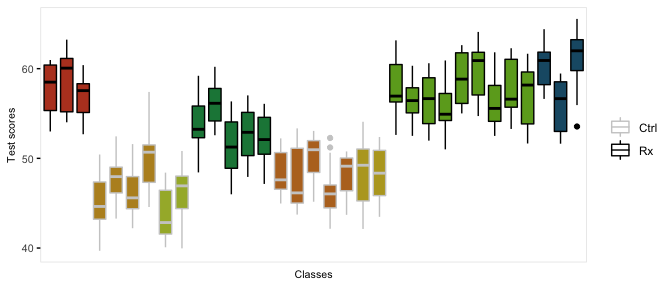## Correlated Data

Sometimes it is desirable to simulate correlated data from a correlation matrix directly. For example, a simulation might require two random effects (e.g. a random intercept and a random slope). Correlated data like this could be generated using the defData functionality, but it may be more natural to do this with genCorData or addCorData. Currently, simstudy can only generate multivariate normal using these functions. (In the future, additional distributions will be available.)

genCorData requires the user to specify a mean vector mu, a single standard deviation or a vector of standard deviations sigma, and either a correlation matrix corMatrix or a correlation coefficient rho and a correlation structure corsrt. It is easy to see how this can be used from a few different examples.

# specifying a specific correlation matrix C
C <- matrix(c(1, 0.7, 0.2, 0.7, 1, 0.8, 0.2, 0.8, 1), nrow = 3)
C
##      [,1] [,2] [,3]
## [1,]  1.0  0.7  0.2
## [2,]  0.7  1.0  0.8
## [3,]  0.2  0.8  1.0
# generate 3 correlated variables with different location and scale for each
# field
dt <- genCorData(1000, mu = c(4, 12, 3), sigma = c(1, 2, 3), corMatrix = C)
dt
##         id       V1        V2         V3
##    1:    1 5.515770 12.144383 -1.0686236
##    2:    2 5.092830 13.703161  4.1447997
##    3:    3 4.643332 13.549768  3.4656835
##    4:    4 3.485077  9.955196 -0.8050064
##    5:    5 3.156134 10.805514  4.2046570
##   ---
##  996:  996 3.061118 10.908654  3.3652916
##  997:  997 4.737585 12.276977 -0.6958394
##  998:  998 6.068601 14.517016  2.4891432
##  999:  999 3.776756 11.568080  1.0854348
## 1000: 1000 3.476381 11.153151  1.9843143
# estimate correlation matrix
dt[, round(cor(cbind(V1, V2, V3)), 1)]
##     V1  V2  V3
## V1 1.0 0.7 0.2
## V2 0.7 1.0 0.8
## V3 0.2 0.8 1.0
# estimate standard deviation
dt[, round(sqrt(diag(var(cbind(V1, V2, V3)))), 1)]
##  V1  V2  V3
## 1.0 2.1 3.1
# generate 3 correlated variables with different location but same standard
# deviation and compound symmetry (cs) correlation matrix with correlation
# coefficient = 0.4.  Other correlation matrix structures are 'independent'
# ('ind') and 'auto-regressive' ('ar1').

dt <- genCorData(1000, mu = c(4, 12, 3), sigma = 3, rho = 0.4, corstr = "cs",
cnames = c("x0", "x1", "x2"))
dt
##         id        x0        x1        x2
##    1:    1 6.7463760  9.187296 0.9490571
##    2:    2 5.0991367 17.028982 7.5477114
##    3:    3 1.4027558  7.762128 3.5508159
##    4:    4 5.4473511 14.240869 4.8175713
##    5:    5 7.7023479 12.053977 7.2223415
##   ---
##  996:  996 6.9708488 11.113277 1.2939515
##  997:  997 4.8871693 11.311965 0.0655096
##  998:  998 6.4291828 12.704555 4.1812567
##  999:  999 0.5680419  6.651471 1.4084934
## 1000: 1000 4.3133160  9.309205 3.8075081
# estimate correlation matrix
dt[, round(cor(cbind(x0, x1, x2)), 1)]
##     x0  x1  x2
## x0 1.0 0.4 0.4
## x1 0.4 1.0 0.4
## x2 0.4 0.4 1.0
# estimate standard deviation
dt[, round(sqrt(diag(var(cbind(x0, x1, x2)))), 1)]
##  x0  x1  x2
## 2.9 3.0 3.0

The new data generated by genCorData can be merged with an existing data set. Alternatively, addCorData will do this directly:

# define and generate the original data set
def <- defData(varname = "x", dist = "normal", formula = 0, variance = 1, id = "cid")
dt <- genData(1000, def)

# add new correlate fields a0 and a1 to 'dt'
dt <- addCorData(dt, idname = "cid", mu = c(0, 0), sigma = c(2, 0.2), rho = -0.2,
corstr = "cs", cnames = c("a0", "a1"))

dt
##        cid            x          a0          a1
##    1:    1  0.004601245 -0.68920187  0.05300875
##    2:    2  0.620824994  2.43418422 -0.11666803
##    3:    3  0.948382872  0.07750747  0.17137369
##    4:    4  1.139409303  5.72105566  0.08655693
##    5:    5  1.291387888  2.60978832  0.06303002
##   ---
##  996:  996  1.502002843  1.27922183  0.04110045
##  997:  997  1.826687943 -0.49886681  0.13734001
##  998:  998  1.635678507 -5.58208426  0.01294041
##  999:  999 -1.469784607  0.48965981 -0.01014777
## 1000: 1000  1.911623120  0.83177201 -0.10237109
# estimate correlation matrix
dt[, round(cor(cbind(a0, a1)), 1)]
##      a0   a1
## a0  1.0 -0.2
## a1 -0.2  1.0
# estimate standard deviation
dt[, round(sqrt(diag(var(cbind(a0, a1)))), 1)]
##  a0  a1
## 2.0 0.2

### Correlated data: additional distributions

Two additional functions facilitate the generation of correlated data from binomial, poisson, gamma, and uniform distributions: genCorGen and addCorGen.

genCorGen is an extension of genCorData. In the first example, we are generating data from a multivariate Poisson distribution. We start by specifying the mean of the Poisson distribution for each new variable, and then we specify the correlation structure, just as we did with the normal distribution.

l <- c(8, 10, 12)  # lambda for each new variable

dx <- genCorGen(1000, nvars = 3, params1 = l, dist = "poisson", rho = 0.3, corstr = "cs",
wide = TRUE)
dx
##         id V1 V2 V3
##    1:    1 11  8  9
##    2:    2  4  8 12
##    3:    3  9 15 13
##    4:    4 11 11 25
##    5:    5  6 10 15
##   ---
##  996:  996 15 10 15
##  997:  997  5 15 12
##  998:  998  6 10 14
##  999:  999  9 11 21
## 1000: 1000 11 16 16
round(cor(as.matrix(dx[, .(V1, V2, V3)])), 2)
##      V1   V2   V3
## V1 1.00 0.26 0.26
## V2 0.26 1.00 0.34
## V3 0.26 0.34 1.00

We can also generate correlated binary data by specifying the probabilities:

genCorGen(1000, nvars = 3, params1 = c(0.3, 0.5, 0.7), dist = "binary", rho = 0.8,
corstr = "cs", wide = TRUE)
##         id V1 V2 V3
##    1:    1  0  1  1
##    2:    2  0  1  1
##    3:    3  0  0  0
##    4:    4  1  1  1
##    5:    5  0  1  1
##   ---
##  996:  996  0  1  1
##  997:  997  0  0  1
##  998:  998  0  0  0
##  999:  999  0  0  0
## 1000: 1000  1  1  1

The gamma distribution requires two parameters - the mean and dispersion. (These are converted into shape and rate parameters more commonly used.)

dx <- genCorGen(1000, nvars = 3, params1 = l, params2 = c(1, 1, 1), dist = "gamma",
rho = 0.7, corstr = "cs", wide = TRUE, cnames = "a, b, c")
dx
##         id          a          b          c
##    1:    1  0.3506233  0.2586153  0.8618973
##    2:    2  9.6212125  8.5800118 10.6524002
##    3:    3  0.1786540  0.6588596  0.1328869
##    4:    4  6.3280989  3.9246574  6.0452989
##    5:    5 13.9802787 24.1283237 17.4796915
##   ---
##  996:  996  4.1634505 19.1533243  8.3935934
##  997:  997  6.8151776 12.7280148  9.1621199
##  998:  998  0.6047445  0.8156330  1.9492700
##  999:  999  1.1640280  3.0878553  5.6904325
## 1000: 1000 14.5295774 11.3923362 22.6556590
round(cor(as.matrix(dx[, .(a, b, c)])), 2)
##      a    b    c
## a 1.00 0.62 0.66
## b 0.62 1.00 0.63
## c 0.66 0.63 1.00

These data sets can be generated in either wide or long form. So far, we have generated wide form data, where there is one row per unique id. Now, we will generate data using the long form, where the correlated data are on different rows, so that there are repeated measurements for each id. An id will have multiple records (i.e. one id will appear on multiple rows):

dx <- genCorGen(1000, nvars = 3, params1 = l, params2 = c(1, 1, 1), dist = "gamma",
rho = 0.7, corstr = "cs", wide = FALSE, cnames = "NewCol")
dx
##         id period    NewCol
##    1:    1      0  2.220021
##    2:    1      1 20.146708
##    3:    1      2  5.232754
##    4:    2      0 10.967238
##    5:    2      1  4.621803
##   ---
## 2996:  999      1  2.012547
## 2997:  999      2  6.988799
## 2998: 1000      0 16.875770
## 2999: 1000      1  2.477952
## 3000: 1000      2 15.286977

addCorGen allows us to create correlated data from an existing data set, as one can already do using addCorData. In the case of addCorGen, the parameter(s) used to define the distribution are created as a field (or fields) in the dataset. The correlated data are added to the existing data set. In the example below, we are going to generate three sets (poisson, binary, and gamma) of correlated data with means that are a function of the variable xbase, which varies by id.

First we define the data and generate a data set:

def <- defData(varname = "xbase", formula = 5, variance = 0.2, dist = "gamma",
id = "cid")
def <- defData(def, varname = "lambda", formula = ".5 + .1*xbase", dist = "nonrandom",
def <- defData(def, varname = "p", formula = "-2 + .3*xbase", dist = "nonrandom",
def <- defData(def, varname = "gammaMu", formula = ".5 + .2*xbase", dist = "nonrandom",
def <- defData(def, varname = "gammaDis", formula = 1, dist = "nonrandom")

dt <- genData(10000, def)
dt
##          cid     xbase   lambda         p   gammaMu gammaDis
##     1:     1  5.735956 2.925881 0.4306467  5.192375        1
##     2:     2  3.004495 2.226542 0.2499927  3.006868        1
##     3:     3 11.731599 5.328980 0.8204618 17.224276        1
##     4:     4  4.182554 2.504917 0.3218607  3.805742        1
##     5:     5  3.007821 2.227282 0.2501798  3.008869        1
##    ---
##  9996:  9996  5.632807 2.895856 0.4230762  5.086355        1
##  9997:  9997  4.555809 2.600181 0.3467723  4.100717        1
##  9998:  9998  2.036695 2.021156 0.1995688  2.477721        1
##  9999:  9999  3.113665 2.250982 0.2561835  3.073242        1
## 10000: 10000  9.686907 4.343544 0.7121957 11.443036        1

The Poisson distribution has a single parameter, lambda:

dtX1 <- addCorGen(dtOld = dt, idvar = "cid", nvars = 3, rho = 0.1, corstr = "cs",
dist = "poisson", param1 = "lambda", cnames = "a, b, c")
dtX1
##          cid seq X a b c
##     1:     1  V1 3 3 3 2
##     2:     1  V2 3 3 3 2
##     3:     1  V3 2 3 3 2
##     4:     2  V1 0 0 2 1
##     5:     2  V2 2 0 2 1
##    ---
## 29996:  9999  V2 2 2 2 2
## 29997:  9999  V3 2 2 2 2
## 29998: 10000  V1 3 3 1 1
## 29999: 10000  V2 1 3 1 1
## 30000: 10000  V3 1 3 1 1

The Bernoulli (binary) distribution has a single parameter, p:

dtX2 <- addCorGen(dtOld = dt, idvar = "cid", nvars = 4, rho = 0.4, corstr = "ar1",
dist = "binary", param1 = "p")
dtX2
##          cid seq X V1 V2 V3 V4
##     1:     1  V1 0  0  0  1  0
##     2:     1  V2 0  0  0  1  0
##     3:     1  V3 1  0  0  1  0
##     4:     1  V4 0  0  0  1  0
##     5:     2  V1 0  0  0  0  0
##    ---
## 39996:  9999  V4 1  0  0  0  1
## 39997: 10000  V1 1  1  1  1  1
## 39998: 10000  V2 1  1  1  1  1
## 39999: 10000  V3 1  1  1  1  1
## 40000: 10000  V4 1  1  1  1  1

The Gamma distribution has two parameters - in simstudy the mean and dispersion are specified:

dtX3 <- addCorGen(dtOld = dt, idvar = "cid", nvars = 4, rho = 0.4, corstr = "cs",
dist = "gamma", param1 = "gammaMu", param2 = "gammaDis")
dtX3
##          cid seq         X        V1        V2        V3        V4
##     1:     1  V1 0.4609004 0.4609004 0.6522854 0.6593119 0.1448655
##     2:     1  V2 0.6522854 0.4609004 0.6522854 0.6593119 0.1448655
##     3:     1  V3 0.6593119 0.4609004 0.6522854 0.6593119 0.1448655
##     4:     1  V4 0.1448655 0.4609004 0.6522854 0.6593119 0.1448655
##     5:     2  V1 0.1735256 0.1735256 2.5185695 1.7314554 2.5526697
##    ---
## 39996:  9999  V4 5.1110858 1.1565474 3.7432964 3.3344199 5.1110858
## 39997: 10000  V1 3.3093331 3.3093331 5.2974786 1.7462736 3.8425898
## 39998: 10000  V2 5.2974786 3.3093331 5.2974786 1.7462736 3.8425898
## 39999: 10000  V3 1.7462736 3.3093331 5.2974786 1.7462736 3.8425898
## 40000: 10000  V4 3.8425898 3.3093331 5.2974786 1.7462736 3.8425898

If we have data in long form (e.g. longitudinal data), the function will recognize the structure:

def <- defData(varname = "xbase", formula = 5, variance = 0.4, dist = "gamma",
id = "cid")
def <- defData(def, "nperiods", formula = 3, dist = "noZeroPoisson")

def2 <- defDataAdd(varname = "lambda", formula = ".5+.5*period + .1*xbase",
dist = "nonrandom", link = "log")

dt <- genData(1000, def)

dtLong <- addPeriods(dt, idvars = "cid", nPeriods = 3)
dtLong <- addColumns(def2, dtLong)

dtLong
##        cid period     xbase nperiods timeID    lambda
##    1:    1      0  3.273140        5      1  2.287167
##    2:    1      1  3.273140        5      2  3.770901
##    3:    1      2  3.273140        5      3  6.217165
##    4:    2      0  2.522111        2      4  2.121686
##    5:    2      1  2.522111        2      5  3.498069
##   ---
## 2996:  999      1 11.098453        4   2996  8.246965
## 2997:  999      2 11.098453        4   2997 13.596947
## 2998: 1000      0 10.832752        6   2998  4.870883
## 2999: 1000      1 10.832752        6   2999  8.030728
## 3000: 1000      2 10.832752        6   3000 13.240433
### Generate the data

dtX3 <- addCorGen(dtOld = dtLong, idvar = "cid", nvars = 3, rho = 0.6, corstr = "cs",
dist = "poisson", param1 = "lambda", cnames = "NewPois")
dtX3
##        cid period     xbase nperiods timeID    param1          U seq
##    1:    1      0  3.273140        5      1  2.287167 0.02481793  V1
##    2:    1      1  3.273140        5      2  3.770901 0.05438466  V2
##    3:    1      2  3.273140        5      3  6.217165 0.18669861  V3
##    4:    2      0  2.522111        2      4  2.121686 0.17547103  V1
##    5:    2      1  2.522111        2      5  3.498069 0.16893248  V2
##   ---
## 2996:  999      1 11.098453        4   2996  8.246965 0.83912046  V2
## 2997:  999      2 11.098453        4   2997 13.596947 0.61508393  V3
## 2998: 1000      0 10.832752        6   2998  4.870883 0.84107217  V1
## 2999: 1000      1 10.832752        6   2999  8.030728 0.84945472  V2
## 3000: 1000      2 10.832752        6   3000 13.240433 0.76717888  V3
##       NewPois i.X
##    1:       0   0
##    2:       1   1
##    3:       4   4
##    4:       1   1
##    5:       2   2
##   ---
## 2996:      11  11
## 2997:      15  15
## 2998:       7   7
## 2999:      11  11
## 3000:      16  16

We can fit a generalized estimating equation (GEE) model and examine the coefficients and the working correlation matrix. They match closely to the data generating parameters:

geefit <- gee(NewPois ~ period + xbase, data = dtX3, id = cid, family = poisson,
corstr = "exchangeable")
## Beginning Cgee S-function, @(#) geeformula.q 4.13 98/01/27
## running glm to get initial regression estimate
## (Intercept)      period       xbase
##  0.52144506  0.50812832  0.09588559
round(summary(geefit)$working.correlation, 2) ## [,1] [,2] [,3] ## [1,] 1.00 0.59 0.59 ## [2,] 0.59 1.00 0.59 ## [3,] 0.59 0.59 1.00 ## Missing Data After generating a complete data set, it is possible to generate missing data. defMiss defines the parameters of missingness. genMiss generates a missing data matrix of indicators for each field. Indicators are set to 1 if the data are missing for a subject, 0 otherwise. genObs creates a data set that reflects what would have been observed had data been missing; this is a replicate of the original data set with “NAs” replacing values where missing data has been generated. By controlling the parameters of missingness, it is possible to represent different missing data mechanisms: (1) missing completely at random (MCAR), where the probability missing data is independent of any covariates, measured or unmeasured, that are associated with the measure, (2) missing at random (MAR), where the probability of subject missing data is a function only of observed covariates that are associated with the measure, and (3) not missing at random (NMAR), where the probability of missing data is related to unmeasured covariates that are associated with measure. These possibilities are illustrated with an example. A data set of 1000 observations with three “outcome” measures" x1, x2, and x3 is defined. This data set also includes two independent predictors, m and u that largely determine the value of each outcome (subject to random noise). def1 <- defData(varname = "m", dist = "binary", formula = 0.5) def1 <- defData(def1, "u", dist = "binary", formula = 0.5) def1 <- defData(def1, "x1", dist = "normal", formula = "20*m + 20*u", variance = 2) def1 <- defData(def1, "x2", dist = "normal", formula = "20*m + 20*u", variance = 2) def1 <- defData(def1, "x3", dist = "normal", formula = "20*m + 20*u", variance = 2) dtAct <- genData(1000, def1) In this example, the missing data mechanism is different for each outcome. As defined below, missingness for x1 is MCAR, since the probability of missing is fixed. Missingness for x2 is MAR, since missingness is a function of m, a measured predictor of x2. And missingness for x3 is NMAR, since the probability of missing is dependent on u, an unmeasured predictor of x3: defM <- defMiss(varname = "x1", formula = 0.15, logit.link = FALSE) defM <- defMiss(defM, varname = "x2", formula = ".05 + m * 0.25", logit.link = FALSE) defM <- defMiss(defM, varname = "x3", formula = ".05 + u * 0.25", logit.link = FALSE) defM <- defMiss(defM, varname = "u", formula = 1, logit.link = FALSE) # not observed missMat <- genMiss(dtName = dtAct, missDefs = defM, idvars = "id") dtObs <- genObs(dtAct, missMat, idvars = "id") missMat ## id x1 x2 x3 u m ## 1: 1 0 1 0 1 0 ## 2: 2 0 1 0 1 0 ## 3: 3 0 0 1 1 0 ## 4: 4 0 0 0 1 0 ## 5: 5 0 0 1 1 0 ## --- ## 996: 996 0 1 0 1 0 ## 997: 997 0 0 1 1 0 ## 998: 998 0 0 0 1 0 ## 999: 999 0 0 0 1 0 ## 1000: 1000 0 0 0 1 0 dtObs ## id m u x1 x2 x3 ## 1: 1 1 NA 40.5775383 NA 39.690947 ## 2: 2 1 NA 18.6875507 NA 21.156386 ## 3: 3 0 NA 1.0101119 -0.4381724 NA ## 4: 4 0 NA -0.6282889 0.2591175 -1.356419 ## 5: 5 0 NA 17.8820291 19.8875343 NA ## --- ## 996: 996 1 NA 19.1160398 NA 19.530451 ## 997: 997 0 NA 20.1203278 19.7550044 NA ## 998: 998 1 NA 20.6368801 20.5669184 18.530801 ## 999: 999 1 NA 16.9314439 19.8567019 24.063291 ## 1000: 1000 1 NA 15.9702069 21.4983511 18.584373 The impacts of the various data mechanisms on estimation can be seen with a simple calculation of means using both the “true” data set without missing data as a comparison for the “observed” data set. Since x1 is MCAR, the averages for both data sets are roughly equivalent. However, we can see below that estimates for x2 and x3 are biased, as the difference between observed and actual is not close to 0: # Two functions to calculate means and compare them rmean <- function(var, digits = 1) { round(mean(var, na.rm = TRUE), digits) } showDif <- function(dt1, dt2, rowName = c("Actual", "Observed", "Difference")) { dt <- data.frame(rbind(dt1, dt2, dt1 - dt2)) rownames(dt) <- rowName return(dt) } # data.table functionality to estimate means for each data set meanAct <- dtAct[, .(x1 = rmean(x1), x2 = rmean(x2), x3 = rmean(x3))] meanObs <- dtObs[, .(x1 = rmean(x1), x2 = rmean(x2), x3 = rmean(x3))] showDif(meanAct, meanObs) ## x1 x2 x3 ## Actual 20.2 20.3 20.2 ## Observed 20.0 19.1 18.6 ## Difference 0.2 1.2 1.6 After adjusting for the measured covariate m, the bias for the estimate of the mean of x2 is mitigated, but not for x3, since u is not observed: meanActm <- dtAct[, .(x1 = rmean(x1), x2 = rmean(x2), x3 = rmean(x3)), keyby = m] meanObsm <- dtObs[, .(x1 = rmean(x1), x2 = rmean(x2), x3 = rmean(x3)), keyby = m] # compare observed and actual when m = 0 showDif(meanActm[m == 0, .(x1, x2, x3)], meanObsm[m == 0, .(x1, x2, x3)]) ## x1 x2 x3 ## Actual 10.4 10.4 10.2 ## Observed 10.6 10.4 8.5 ## Difference -0.2 0.0 1.7 # compare observed and actual when m = 1 showDif(meanActm[m == 1, .(x1, x2, x3)], meanObsm[m == 1, .(x1, x2, x3)]) ## x1 x2 x3 ## Actual 29.4 29.5 29.4 ## Observed 29.0 30.0 28.0 ## Difference 0.4 -0.5 1.4 ## Longitudinal Data with Missingness Missingness can occur, of course, in the context of longitudinal data. missDef provides two additional arguments that are relevant for these types of data: baseline and monotonic. In the case of variables that are measured at baseline only, a missing value would be reflected throughout the course of the study. In the case where a variable is time-dependent (i.e it is measured at each time point), it is possible to declare missingness to be monotonic. This means that if a value for this field is missing at time t, then values will also be missing at all times T > t as well. The call to genMiss must set repeated to TRUE. The following two examples describe an outcome variable y that is measured over time, whose value is a function of time and an observed exposure: # use baseline definitions from previous example dtAct <- genData(120, def1) dtAct <- trtObserve(dtAct, formulas = 0.5, logit.link = FALSE, grpName = "rx") # add longitudinal data defLong <- defDataAdd(varname = "y", dist = "normal", formula = "10 + period*2 + 2 * rx", variance = 2) dtTime <- addPeriods(dtAct, nPeriods = 4) dtTime <- addColumns(defLong, dtTime) In the first case, missingness is not monotonic; a subject might miss a measurement but returns for subsequent measurements: # missingness for y is not monotonic defMlong <- defMiss(varname = "x1", formula = 0.2, baseline = TRUE) defMlong <- defMiss(defMlong, varname = "y", formula = "-1.5 - 1.5 * rx + .25*period", logit.link = TRUE, baseline = FALSE, monotonic = FALSE) missMatLong <- genMiss(dtName = dtTime, missDefs = defMlong, idvars = c("id", "rx"), repeated = TRUE, periodvar = "period") Here is a conceptual plot that shows the pattern of missingness. Each row represents an individual, and each box represents a time period. A box that is colored reflects missing data; a box colored grey reflects observed. The missingness pattern is shown for two variables x1 and y: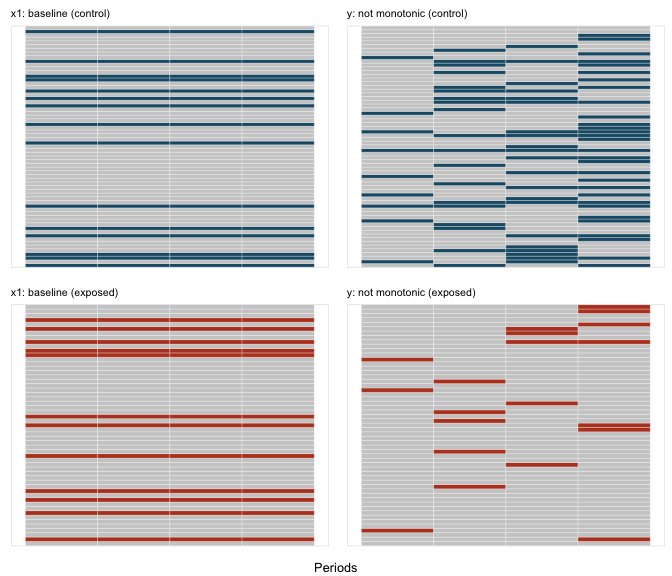In the second case, missingness is monotonic; once a subject misses a measurement for y, there are no subsequent measurements: # missingness for y is not monotonic defMlong <- defMiss(varname = "x1", formula = 0.2, baseline = TRUE) defMlong <- defMiss(defMlong, varname = "y", formula = "-1.8 - 1.5 * rx + .25*period", logit.link = TRUE, baseline = FALSE, monotonic = TRUE) missMatLong <- genMiss(dtName = dtTime, missDefs = defMlong, idvars = c("id", "rx"), repeated = TRUE, periodvar = "period")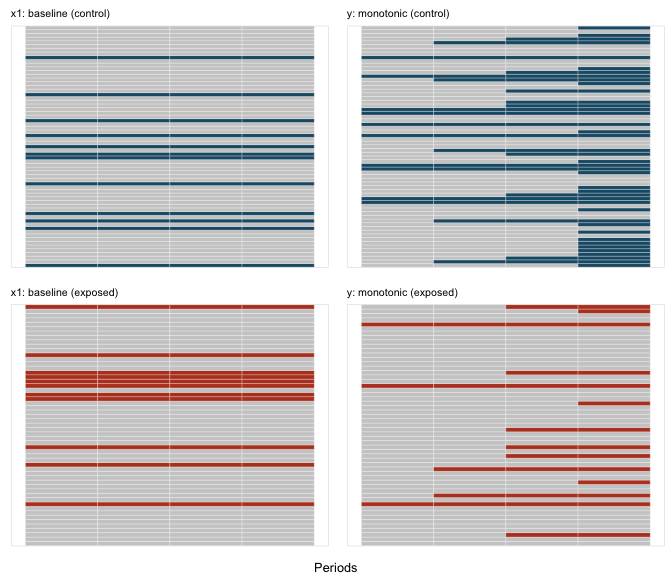## Spline Data Sometimes (usually?) relationships between variables are non-linear. simstudy can already accommodate that. But, if we want to explicitly generate data from a piece-wise polynomial function to explore spline methods in particular, or non-linear relationships more generally. There are three functions that facilitate this: viewBasis, viewSplines, and genSpline. The first two functions are more exploratory in nature, and just provide plots of the B-spline basis functions and the splines, respectively. The third function actually generates data and adds to an existing data.table. The shape of a spline is determined by three factors: (1) the cut-points or knots that define the piecewise structure of the function, (2) the polynomial degree, such as linear, quadratic, cubic, etc., and (3) the linear coefficients that combine the basis functions, which is contained in a vector or matrix theta. First, we can look at the basis functions, we depend only the knots and degree. The knots are specified as quantiles, between 0 and 1: knots <- c(0.25, 0.5, 0.75) viewBasis(knots, degree = 2)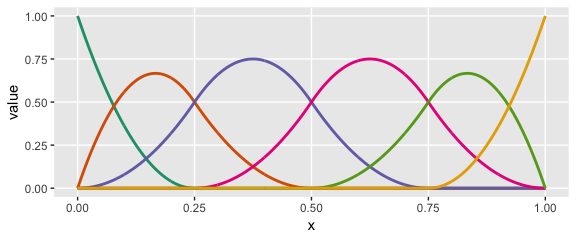knots <- c(0.2, 0.4, 0.6, 0.8) viewBasis(knots, degree = 3)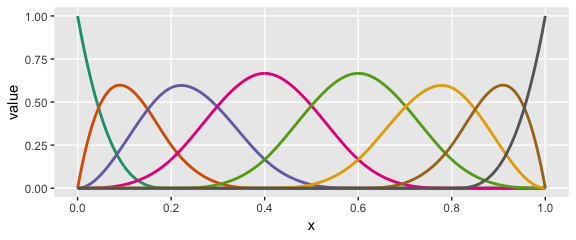The splines themselves are specified as linear combinations of each of the basis functions. The coefficients of those combinations are specified in theta. Each individual spline curve represents a specific linear combination of a particular set of basis functions. In exploring, we can look at a single curve or multiple curves, depending on whether or not we specify theta as a vector (single) or matrix (multiple). knots <- c(0.25, 0.5, 0.75) # number of elements in theta: length(knots) + degree + 1 theta1 = c(0.1, 0.8, 0.4, 0.9, 0.2, 1) viewSplines(knots, degree = 2, theta1)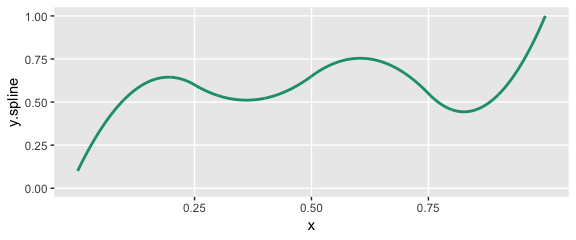theta2 = matrix(c(0.1, 0.2, 0.4, 0.9, 0.2, 0.3, 0.6, 0.1, 0.3, 0.3, 0.8, 1, 0.9, 0.4, 0.1, 0.9, 0.8, 0.2, 0.1, 0.6, 0.1), ncol = 3) theta2 ## [,1] [,2] [,3] ## [1,] 0.1 0.1 0.1 ## [2,] 0.2 0.3 0.9 ## [3,] 0.4 0.3 0.8 ## [4,] 0.9 0.8 0.2 ## [5,] 0.2 1.0 0.1 ## [6,] 0.3 0.9 0.6 ## [7,] 0.6 0.4 0.1 viewSplines(knots, degree = 3, theta2)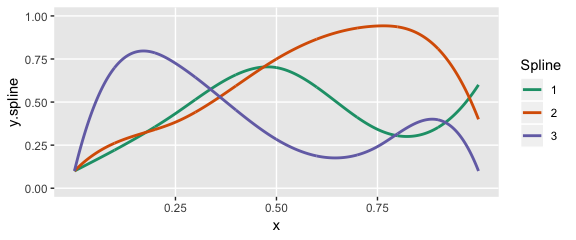We can generate data using a predictor in an existing data set by specifying the knots (in terms of quantiles), a vector of coefficients in theta, the degree of polynomial, as well as a range ddef <- defData(varname = "age", formula = "20;60", dist = "uniform") theta1 = c(0.1, 0.8, 0.6, 0.4, 0.6, 0.9, 0.9) knots <- c(0.25, 0.5, 0.75) Here is the shape of the curve that we want to generate data from: viewSplines(knots = knots, theta = theta1, degree = 3)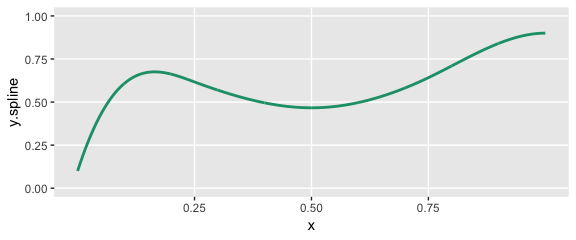Now we specify the variables in the data set and generate the data: set.seed(234) dt <- genData(1000, ddef) dt <- genSpline(dt = dt, newvar = "weight", predictor = "age", theta = theta1, knots = knots, degree = 3, newrange = "90;160", noise.var = 64) Here’s a plot of the data with a smoothed line fit to the data: ggplot(data = dt, aes(x = age, y = weight)) + geom_point(color = "grey65", size = 0.75) + geom_smooth(se = FALSE, color = "red", size = 1, method = "auto") + geom_vline(xintercept = quantile(dt$age,
knots)) + theme(panel.grid.minor = element_blank())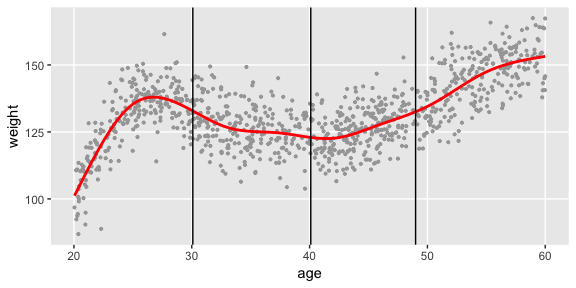Finally, we will fit three different spline models to the data - a linear, a quadratic, and a cubic - and plot the predicted values:

# normalize age for best basis functions
dt[, :=(nage, (age - min(age))/(max(age) - min(age)))]

# fit a cubic spline
lmfit3 <- lm(weight ~ bs(x = nage, knots = knots, degree = 3, intercept = TRUE) -
1, data = dt)

# fit a quadtratic spline
lmfit2 <- lm(weight ~ bs(x = nage, knots = knots, degree = 2), data = dt)

# fit a linear spline
lmfit1 <- lm(weight ~ bs(x = nage, knots = knots, degree = 1), data = dt)

# add predicted values for plotting
dt[, :=(pred.3deg, predict(lmfit3))]
dt[, :=(pred.2deg, predict(lmfit2))]
dt[, :=(pred.1deg, predict(lmfit1))]

ggplot(data = dt, aes(x = age, y = weight)) + geom_point(color = "grey65", size = 0.75) +
geom_line(aes(x = age, y = pred.3deg), color = "#1B9E77", size = 1) + geom_line(aes(x = age,
y = pred.2deg), color = "#D95F02", size = 1) + geom_line(aes(x = age, y = pred.1deg),
color = "#7570B3", size = 1) + geom_vline(xintercept = quantile(dt\$age,
knots)) + theme(panel.grid.minor = element_blank())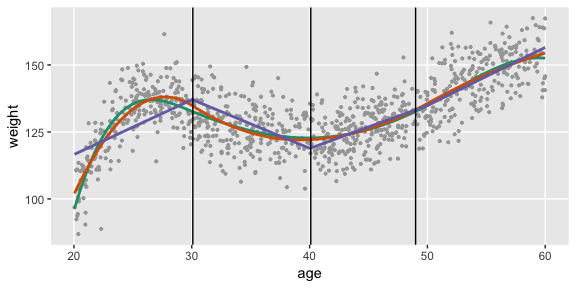## Ordinal Categorical Data

Using the defData and genData functions, it is is relatively easy to specify multinomial distributions that characterize categorical data. Order becomes relevant when the categories take on meanings related strength of opinion or agreement (as in a Likert-type response) or frequency. A motivating example could be when a response variable takes on five possible values: (1) strongly disagree, (2) disagree, (3) neutral, (4) agree, (5) strongly agree. There is a natural order to the response possibilities.

It is common to summarize the data by looking at cumulative probabilities, odds, or log-odds. Comparisons of different exposures or individual characteristics typically look at how these cumulative measures vary across the different exposures or characteristics. So, if we were interested in cumulative odds, we would compare $\small{\frac{P(response = 1|exposed)}{P(response > 1|exposed)} \ \ vs. \ \frac{P(response = 1|unexposed)}{P(response > 1|unexposed)}},$

$\small{\frac{P(response \le 2|exposed)}{P(response > 2|exposed)} \ \ vs. \ \frac{P(response \le 2|unexposed)}{P(response > 2|unexposed)}},$

and continue until the last (in this case, fourth) comparison

$\small{\frac{P(response \le 4|exposed)}{P(response > 4|exposed)} \ \ vs. \ \frac{P(response \le 4|unexposed)}{P(response > 4|unexposed)}},$

We can use an underlying (continuous) latent process as the basis for data generation. If we assume that probabilities are determined by segments of a logistic distribution (see below), we can define the ordinal mechanism using thresholds along the support of the distribution. If there are $$k$$ possible responses (in the meat example, we have 5), then there will be $$k-1$$ thresholds. The area under the logistic density curve of each of the regions defined by those thresholds (there will be $$k$$ distinct regions) represents the probability of each possible response tied to that region.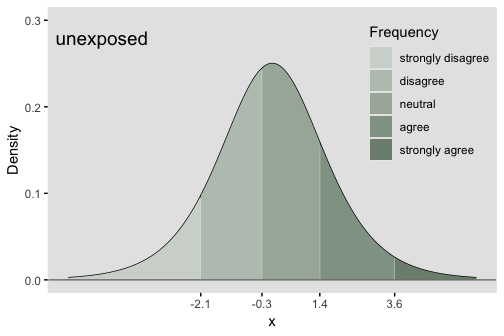### Comparing response distributions of different populations

In the cumulative logit model, the underlying assumption is that the odds ratio of one population relative to another is constant across all the possible responses. This means that all of the cumulative odds ratios are equal:

$\small{\frac{codds(P(Resp = 1 | exposed))}{codds(P(Resp = 1 | unexposed))} = \frac{codds(P(Resp \leq 2 | exposed))}{codds(P(Resp \leq 2 | unexposed))} = \ ... \ = \frac{codds(P(Resp \leq 4 | exposed))}{codds(P(Resp \leq 4 | unexposed))}}$

In terms of the underlying process, this means that each of the thresholds shifts the same amount, as shown below, where we add 1.1 units to each threshold that was set for the exposed group. What this effectively does is create a greater probability of a lower outcome for the unexposed group.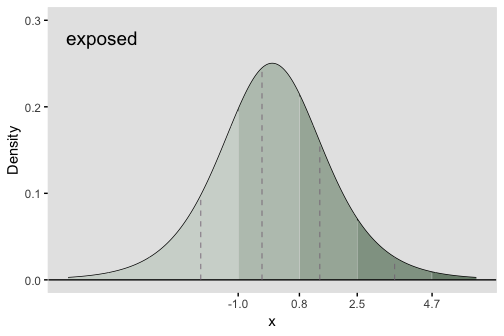### The cumulative proportional odds model

In the R package ordinal, the model is fit using function clm. The model that is being estimated has the form

$log \left( \frac{P(Resp \leq i)}{P(Resp > i)} | Group \right) = \alpha_i - \beta*I(Group=exposed) \ \ , \ i \in \{1, 2, 3, 4\}$

The model specifies that the cumulative log-odds for a particular category is a function of two parameters, $$\alpha_i$$ and $$\beta$$. (Note that in this parameterization and the model fit, $$-\beta$$ is used.) $$\alpha_i$$ represents the cumulative log odds of being in category $$i$$ or lower for those in the reference exposure group, which in our example is Group A. $$\alpha_i$$ also represents the threshold of the latent continuous (logistic) data generating process. $$\beta$$ is the cumulative log-odds ratio for the category $$i$$ comparing the unexposed to reference group, which is the exposed. $$\beta$$ also represents the shift of the threshold on the latent continuous process for the exposed relative to the unexposed. The proportionality assumption implies that the shift of the threshold for each of the categories is identical.

### Simulation

To generate ordered categorical data using simstudy, there is a function genOrdCat.

baseprobs <- c(0.11, 0.33, 0.36, 0.17, 0.03)

defA <- defDataAdd(varname = "z", formula = "-1.1*exposed", dist = "nonrandom")

set.seed(130)

dT <- genData(25000)
dT <- trtAssign(dT, grpName = "exposed")
dT <- addColumns(defA, dT)

dT <- genOrdCat(dT, adjVar = "z", baseprobs, catVar = "r")

Estimating the parameters of the model using function clm, we can recover the original parameters quite well.

clmFit <- clm(r ~ exposed, data = dT)
summary(clmFit)
## formula: r ~ exposed
## data:    dT
##
##  link  threshold nobs  logLik    AIC      niter max.grad cond.H
##  logit flexible  25000 -33309.96 66629.91 6(0)  1.97e-10 2.3e+01
##
## Coefficients:
##         Estimate Std. Error z value Pr(>|z|)
## exposed   -1.119      0.024   -46.5   <2e-16 ***
## ---
## Signif. codes:  0 '***' 0.001 '**' 0.01 '*' 0.05 '.' 0.1 ' ' 1
##
## Threshold coefficients:
##     Estimate Std. Error z value
## 1|2  -2.1066     0.0222   -94.8
## 2|3  -0.2554     0.0173   -14.7
## 3|4   1.3448     0.0203    66.3
## 4|5   3.4619     0.0456    75.9

In the model output, the exposed coefficient of -1.15 is the estimate of $$-\beta$$ (i.e. $$\hat{\beta} = 1.15$$), which was set to -1.1 in the simulation. The threshold coefficients are the estimates of the $$\alpha_i$$’s in the model - and match the thresholds for the unexposed group.

The log of the cumulative odds for groups 1 to 4 from the data without exposure are

(logOdds.unexp <- log(odds(cumsum(dT[exposed == 0, prop.table(table(r))])))[1:4])
##     1     2     3     4
## -2.10 -0.25  1.34  3.46

And under exposure:

(logOdds.expos <- log(odds(cumsum(dT[exposed == 1, prop.table(table(r))])))[1:4])
##     1     2     3     4
## -0.99  0.86  2.49  4.60

The log of the cumulative odds ratios for each of the four groups is

logOdds.expos - logOdds.unexp
##   1   2   3   4
## 1.1 1.1 1.2 1.1

### Correlated multivariate ordinal data

Function genCorOrdCat generates multiple categorical response variables that may be correlated. For example, a survey of multiple Likert-type questions could have many response variables. The function generates correlated latent variables (using a normal copula) to simulate correlated categorical outcomes. The user specifies a matrix of probabilities, with each row representing a single item or categorical variable. The across each row must be 1. Adjustment variables can be specified for each item, or a single adjustment variable can be specified for all items. The correlation is on the standard normal scale, and is specified with a value of rho and a correlation structure (independence, compound symmetry, or AR-1). Alternatively, a correlation matrix can be specified.

In this example, there are 5 questions, each of which has three possible responses: “none”, “some”, “a lot”. The probabilities of response are specified in a $$5 \times 3$$ matrix, and the rows sum to 1:

baseprobs <- matrix(c(0.2, 0.1, 0.7, 0.7, 0.2, 0.1, 0.5, 0.2, 0.3, 0.4, 0.2,
0.4, 0.6, 0.2, 0.2), nrow = 5, byrow = TRUE)

# generate the data

set.seed(333)
dT <- genData(10000)

dX <- genCorOrdCat(dT, adjVar = NULL, baseprobs = baseprobs, prefix = "q", rho = 0.15,
corstr = "cs")

The observed correlation of the items is slightly less than the specified correlations as expected:

round(dX[, cor(cbind(q1, q2, q3, q4, q5))], 2)
##      q1   q2   q3   q4   q5
## q1 1.00 0.08 0.10 0.10 0.08
## q2 0.08 1.00 0.09 0.09 0.09
## q3 0.10 0.09 1.00 0.11 0.11
## q4 0.10 0.09 0.11 1.00 0.10
## q5 0.08 0.09 0.11 0.10 1.00

However, the marginal probability distributions of each item match quite closely with the specified probabilities:

dM <- melt(dX, id.vars = "id")
dProp <- dM[, prop.table(table(value)), by = variable]
dProp[, :=(response, rep(seq(3), 5))]

# observed probabilities
dcast(dProp, variable ~ response, value.var = "V1", fill = 0)
##    variable    1    2    3
## 1:       q1 0.20 0.10 0.70
## 2:       q2 0.69 0.21 0.10
## 3:       q3 0.50 0.20 0.30
## 4:       q4 0.40 0.20 0.40
## 5:       q5 0.60 0.20 0.21
# specified probabilites
baseprobs
##      [,1] [,2] [,3]
## [1,]  0.2  0.1  0.7
## [2,]  0.7  0.2  0.1
## [3,]  0.5  0.2  0.3
## [4,]  0.4  0.2  0.4
## [5,]  0.6  0.2  0.2

In the next example, the structure of the correlation is changed to AR-1, so the correlation between questions closer to each other is higher than for questions farther apart. But the probability distributions are unaffected:

dX <- genCorOrdCat(dT, adjVar = NULL, baseprobs = baseprobs, prefix = "q", rho = 0.4,
corstr = "ar1")

# correlation
round(dX[, cor(cbind(q1, q2, q3, q4, q5))], 2)
##      q1   q2   q3   q4   q5
## q1 1.00 0.22 0.10 0.05 0.02
## q2 0.22 1.00 0.29 0.10 0.03
## q3 0.10 0.29 1.00 0.31 0.11
## q4 0.05 0.10 0.31 1.00 0.29
## q5 0.02 0.03 0.11 0.29 1.00
dM <- melt(dX, id.vars = "id")
dProp <- dM[, prop.table(table(value)), by = variable]
dProp[, :=(response, rep(seq(3), 5))]

# probabilities
dcast(dProp, variable ~ response, value.var = "V1", fill = 0)
##    variable    1    2     3
## 1:       q1 0.20 0.10 0.692
## 2:       q2 0.70 0.20 0.099
## 3:       q3 0.50 0.20 0.300
## 4:       q4 0.39 0.21 0.397
## 5:       q5 0.60 0.19 0.204